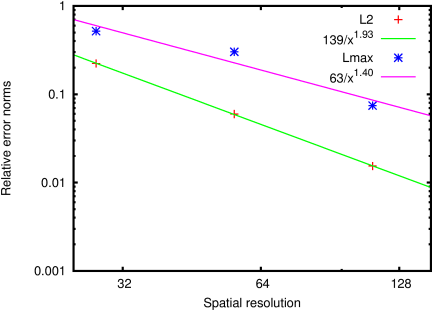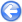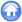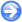### 4.1PASS: Estimation of the numerical viscosity

Author
Stéphane Popinet
Command
sh reynolds.sh reynolds.gfs 1
Version
0.6.4
Required files
reynolds.sh div5.ref div6.ref div7.ref reynolds.ref
Running time
3 minutes 26 seconds

The velocity field is initialised with an exact stationary solution of the Euler equations in a periodic 2D domain. An exact Euler solver would not change this field, however any numerical solver will introduce numerical dissipation which will slowly dissipate the kinetic energy of the initial solution. By monitoring the evolution of the kinetic energy, the dissipative properties of the numerical scheme can be measured (see  for details).

Figures 45 and figure 46 illustrate the evolution of the divergence of the velocity field with time. This is a check of the stability of the approximate projection and should remain bounded.

Figures 47 and 48 illustrates the evolution of the kinetic energy and the corresponding equivalent Reynolds number as a function of resolution. The higher the Reynolds number, the less dissipative the scheme.

 Figure 45: Evolution of the maximum divergence.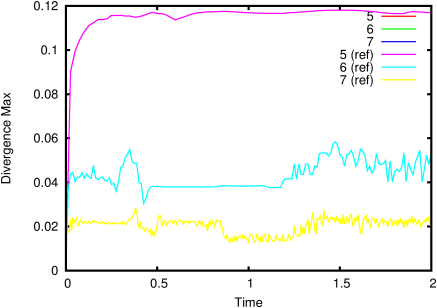Figure 46: Evolution of the L2 norm of the divergence.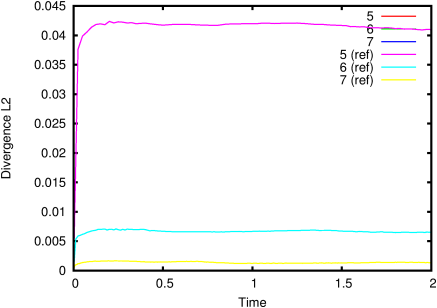Figure 47: Evolution of the kinetic energy.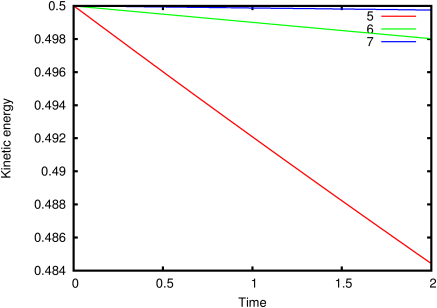Figure 48: Equivalent Reynolds number as a function of resolution.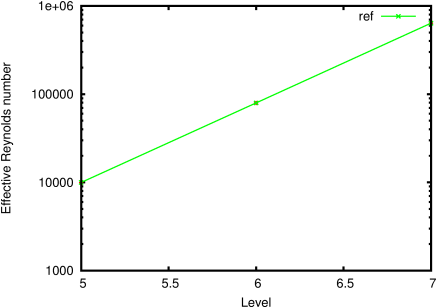Figure 49: Accuracy of the solution as a function of the level of refinement.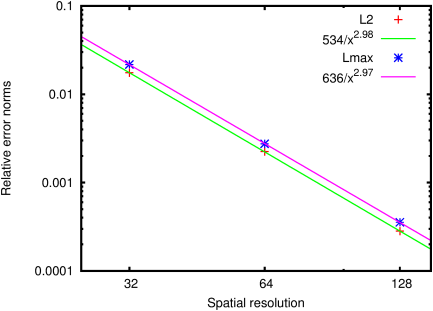#### 4.1.1PASS: Estimation of the numerical viscosity with refined box

Author
Stéphane Popinet
Command
sh ../reynolds.sh box.gfs 4
Version
0.6.4
Required files
../reynolds.sh div5.ref div6.ref div7.ref reynolds.ref
Running time
19 minutes 45 seconds

Same as the previous test but with a refined box in the middle and four modes of the exact Euler solution.

 Figure 50: Evolution of the maximum divergence.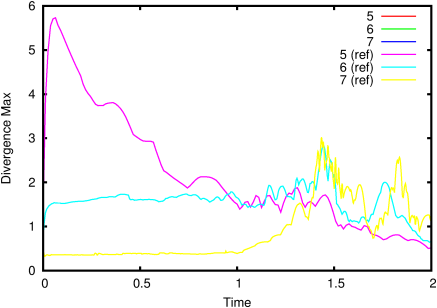Figure 51: Evolution of the L2 norm of the divergence.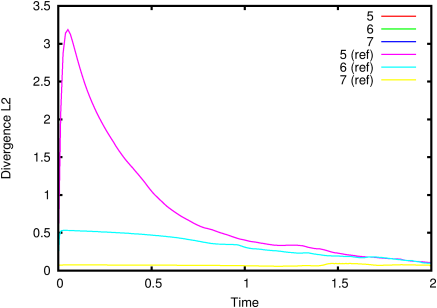Figure 52: Evolution of the kinetic energy.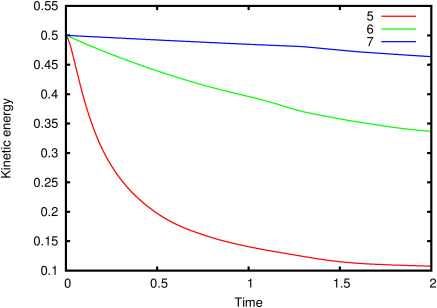Figure 53: Equivalent Reynolds number as a function of resolution.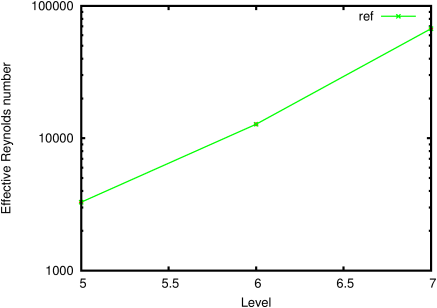Figure 54: Accuracy of the solution as a function of the level of refinement.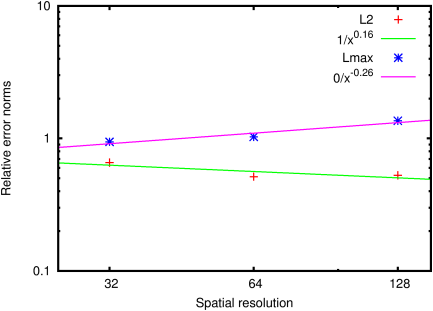#### 4.1.2PASS: Numerical viscosity for vorticity/streamfunction formulation

Author
Stéphane Popinet
Command
sh ../reynolds.sh stream.gfs 1
Version
110610
Required files
div5.ref div6.ref div7.ref reynolds.ref
Running time
1 minutes 27 seconds

Same as the previous test but using a vorticity/streamfunction formulation.

 Figure 55: Evolution of the maximum divergence.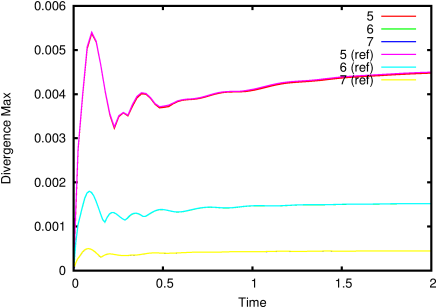Figure 56: Evolution of the L2 norm of the divergence.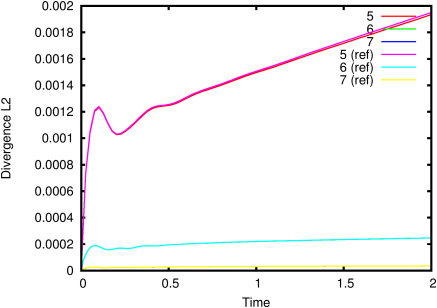Figure 57: Evolution of the kinetic energy.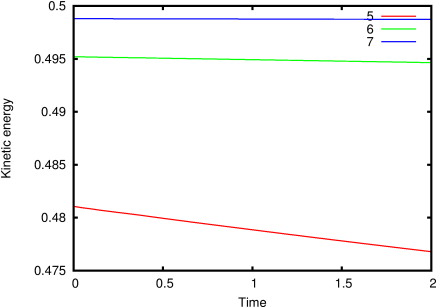Figure 58: Equivalent Reynolds number as a function of resolution.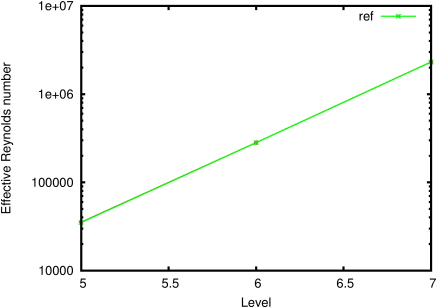Figure 59: Accuracy of the solution as a function of the level of refinement.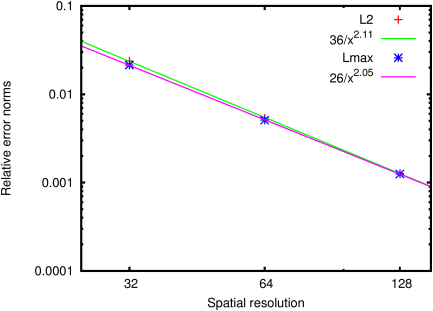#### 4.1.3PASS: Numerical viscosity for the skew-symmetric scheme

Author
Daniel Fuster
Command
sh skew.sh skew.gfs 1
Version
110723
Required files
skew.sh div5.ref div6.ref div7.ref reynolds.ref
Running time
1 minutes 17 seconds

Same as the previous test but using the skew-symmetric module.

 Figure 60: Evolution of the maximum divergence.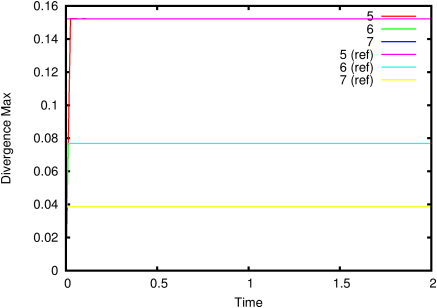Figure 61: Evolution of the L2 norm of the divergence.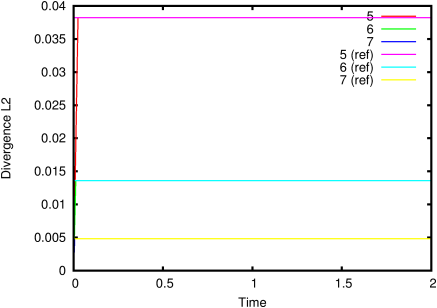Figure 62: Evolution of the kinetic energy.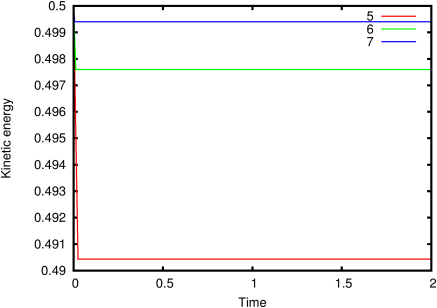Figure 63: Accuracy of the solution as a function of the level of refinement.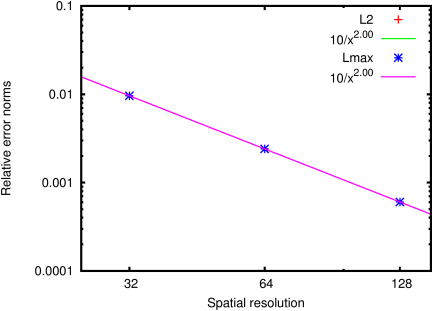#### 4.1.4PASS: Numerical viscosity for the skew-symmetric scheme with refined box

Author
Daniel Fuster
Command
sh skew.sh skewbox.gfs 1
Version
120229
Required files
skew.sh error.ref
Running time
12 minutes 28 seconds

The skew-symmetric module with AMR.

 Figure 64: Evolution of the kinetic energy.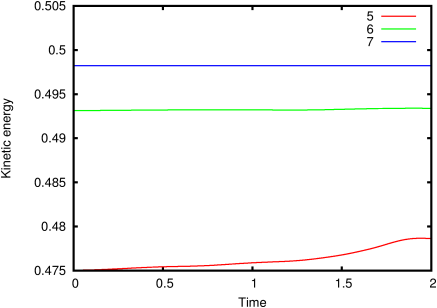Figure 65: Accuracy of the solution as a function of the level of refinement.